StatLect

# Maximum likelihood - MATLAB Example

In the lecture entitled Maximum likelihood - Algorithm we have explained how to compute the maximum likelihood estimator of a parameter by numerical methods. In this lecture we provide a fully worked out example that illustrates how to do so with MATLAB.

In what follows, I will assume that you have access to a MATLAB installation comprising both the Statistics and the Optimization toolboxes (an installation of Octave - a free software that is very similar to MATLAB - should provide the same functionality).## Data

We have a sample of 100 independent draws from a standard Student's t distribution withdegrees of freedom. The parameteris unknown and we want to estimate it by maximum likelihood.

The data (the 100 observations) are stored in the MATLAB file data.mat, which you need to download.

## Parametrization

Note that the parametermust be strictly positive, that is, it must belong to the interval. Therefore, the optimization problem we need to solve in order to estimateis a constrained optimization problem. As explained in the lecture Maximum likelihood - Algorithm, it is preferable to avoid constrained problems when possible. In this case, it is possible becausecan be easily reparametrized as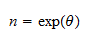whereis our new parameter and there are no constraints on it, because it can take any value in the interval.

## Coding the log-likelihood function

The likelihood function is coded as a routine that takes as inputs a value for the parameter and the data, and returns as output the value of the log-likelihood with its sign changed. The code is as follows.

```function val=log_lik(theta,data)
n=exp(theta);
val=-sum(log(tpdf(data,n)));
```

The name of the function is `log_lik`. It takes as arguments the parameter `theta` and the vector of observations `data`.

The function `tpdf` (which is part of the Statistics toolbox) computes the probability density function of a Standard Student's t distribution. In particular, `tpdf(data,n)` returns a vector of densities (one density for each observation in the vector `data`), under the hypothesis that the number of degrees of freedom is equal to `n`. By taking the natural logarithm with the `log` function and summing over all entries of the vector, we obtain the log-likelihood of the sample. In other words, with the command `sum(log(tpdf(data,df)))` we compute the log-likelihood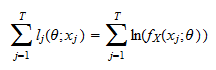whereis an observation (a component of the vector `data`),is the sample size (the dimension of the vector `data`) andis the probability density function of, given that the parameter is equal to.

Finally, we change the sign of the log-likelihood, by putting a minus in front of it, because the optimization routine we are going to use performs minimization by default, and we instead want to maximize the log-likelihood function. The value thus obtained is stored in the variable `val`, which is returned by the function.

## The optimization algorithm

The code presented in this subsection runs the optimization algorithm, in order to find numerically the maximum likelihood estimator of the parameter.

```load data
options=optimset('Display','off','MaxIter',10000,'TolX',10^-30,'TolFun',10^-30);
theta0=1;
exp(theta)
```

We first load the data file with the command ```load data```.

We then set some options of the optimization algorithm. The option `Display` is set to `off`, which means that the optimization algorithm will run silently, without showing the output of each iteration. The option `MaxIter` is set to `10000`, which means that the algorithm will perform a maximum of 10,000 iterations. The option `TolX` is set to `10^-30`, which means that the termination tolerance on the parameter will be. Also the option `TolFun` is set to `10^-30`, which means that the termination tolerance on the value of the function to be optimized will be.

The starting value for the parameter (our initial guess) is set equal to 1 with the command `theta0=1`.

Finally, the MATLAB derivative-based optimization algorithm `fminunc` is called. It takes as arguments:

• the name of the function to be optimized (`log_lik`);

• the initial value of the parameter (`theta0`);

• the options that were previously set (`options`);

• any other arguments besides the parameter that need to be passed to the function to be optimized (in our case the vector `data`).

The function returns as outputs:

• the estimated solution of the minimization problem (`theta`);

• the value of the likelihood-function corresponding to the estimated solution (`val`)

• a number that tells which termination criterion was met when the algorithm stopped (`exitflag`)

• some information about what happened during the optimization process, including the number of iterations that were performed and a verbal description of how the algorithm reached the estimated solution (`output`)

• the value of the gradient at `theta`, that is, the vector of first derivatives of the log-likelihood function, evaluated numerically at the estimated solution (`grad`);

• the value of the Hessian at `theta`, that is, the matrix of second derivatives of the log-likelihood function, evaluated numerically at the estimated solution (`hessian`).

The last line of code (`exp(theta)`) displays the estimate of the parameter, which is equal to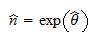whereis the maximum likelihood estimate of(stored in the variable `theta`). If the code is correctly executed, the displayed value should be 3.9389, which is our point estimate of the parameter of interest. This corresponds to an estimate of 1.3709 for.

You can also display the value of the gradient (by typing `grad`). A number that is numerically close to zero should be displayed, which means that the first order condition for an optimum (i.e., first derivative equals zero) is approximately satisfied.

## Multiple starts

We do not know whether the properties of the `fminunc` algorithm, together with the properties of our likelihood function, guarantee numerical convergence to the true solution of the problem. As we have previously explained, when there is no theoretical guarantee that numerical convergence can be achieved, a multiple starts approach is usually followed: the numerical optimization algorithm is run several times, with random starting values for the parameter, and if all runs of the algorithm (or a majority of them) lead to the same proposed solution, then this is taken as evidence that the proposed solution is a good approximation of the true solution.

The following code is a multiple starts variant of the code explained above.

```load data
stream=RandStream('mt19937ar','Seed',1);
RandStream.setDefaultStream(stream);
options=optimset('Display','off','MaxIter',10000,'TolX',10^-30,'TolFun',10^-30);
for j=1:10
theta0=1+10*randn(1,1);
fminunc('log_lik',theta0,options,data);
end
theta
```

The two commands involving `RandStream` initialize the random number generator. You should not worry about these two commands. They are there just to ensure that, if you run this code on your computer, you will get exactly the same results I get.

The optimization is repeated ten times, and each time the starting value `theta0` is generated randomly. In particular, we add to our initial guess (`theta0=1`) a random number extracted from a standard normal distribution (`randn(1,1)`), multiplied by 10.

The outputs are stored in vectors, whose entries are indexed by the variable `j`. In particular, the ten proposed solutions are stored in the vector `theta`, and the ten values of the log-likelihood function corresponding to these solutions are stored in the vector `val`.

If, after running the script, you print the proposed solutions by typing `theta`, you will see that they are all equal to the one we had found previously (1.3709), except for one - the second - which is equal to 12.8117. Thus, we have encountered some trouble. This confirms our suspicion that it was not a wise idea to be satisfied with only one run of the algorithm.

By printing the vectors `val` and `grad`, we can easily see that 12.8117 cannot be a solution, because it corresponds to a value of the objective function that is higher than the value corresponding to 1.3709, and because the gradient is not equal to zero.

If we run the algorithm many more times (e.g., increase the number of repetitions to 1000 in the `for` cycle), we will see that also other solutions are proposed, but they are not really solutions, because of the same reasons (i.e., non-zero derivative, higher value of the objective function).

Thus, we can take the result of the multiple starts algorithm as evidence that 1.3709 is a sound solution. At the same time, this reminds us of the fact that it is really a bad idea to run an optimization algorithm only once if we are not sure of its convergence properties.

## Estimating the variance

To produce an estimate of the variance of, we can use one of the methods introduced in the lecture Maximum likelihood - Covariance matrix estimation. In particular, since `fminunc` provides a numerical estimate of the Hessian matrix, we can use a method based on this estimate.

Remember that the distribution of the maximum likelihood estimatorcan be approximated by a multivariate normal distribution with mean equal to the true parameterand covariance matrix equal towhere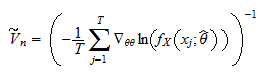is an estimate of the asymptotic covariance matrix anddenotes the matrix of second derivatives. In other words, the estimate of the variance ofis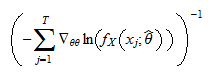In our case there is only one parameter, so the matrix is actually a scalar and we can write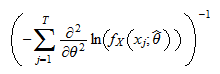The value stored by `fminunc` in the variable `hessian` is the second derivative of `log_lik`, which is (see above)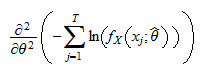By linearity, this is the same as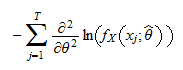Therefore, all we need to do in order to obtain an estimate of the variance ofis to compute the reciprocal of `hessian`, with the command `hessian^-1` (if you do this after running the multiple starts algorithm, take only one entry of the vector `hessian`, corresponding to the estimate 1.3709). If everything was done correctly, the estimate should be equal to 0.0970. This can be translated into an estimate of the variance ofwith the Delta method, by multiplying the estimated variance ofby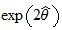. As a result, we obtain that the estimated variance ofis 1.5046.

## MATLAB files

All the MATLAB codes presented in this lecture are stored in a zipped file, which you can download.

The book

Most of the learning materials found on this website are now available in a traditional textbook format.

Glossary entries
Share